International
Tables for
Crystallography
Volume C
Mathematical, physical and chemical tables
Edited by E. Prince

International Tables for Crystallography (2006). Vol. C, ch. 6.4, p. 611

Section 6.4.10.2. The uncorrelated block model

T. M. Sabinea

aANSTO, Private Mail Bag 1, Menai, NSW 2234, Australia

6.4.10.2. The uncorrelated block model

| top | pdf |

When this model is used, two values of x are required. These are designated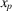for primary extinction and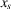for secondary extinction. Equation (6.4.8.1)is used to obtain a value for. The primary-extinction factors are then calculated from (6.4.5.3), (6.4.5.4)and (6.4.5.5), and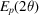is given by equation (6.4.7.1). In the second step,is obtained from equation (6.4.9.1), and the secondary-extinction factors are calculated from either (6.4.9.2)and (6.4.9.3)or (6.4.9.4)and (6.4.9.5). The result of these calculations is then used in equation (6.4.7.1)to give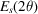. It is emphasised thatincludes the primary-extinction factor. Finally,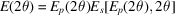.

Application of both models to the analysis of neutron diffraction data has been carried out by Kampermann, Sabine, Craven & McMullan (1995).

References

Kampermann, S. P., Sabine, T. M., Craven, B. M. & McMullan, R. K. (1995). Hexamethylenetetramine: extinction and thermal vibrations from neutron diffraction at six temperatures. Acta Cryst. A51, 489–497.